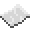# vector.div

Given a vector, self, and a scalar this function will divide each component in self by the scalar to create a new vector. This can also be written as `self / scalar`. If self was not created with vector.new, this function will not work as designed.ExampleDivide a vector
Divide a vector by a number.
Code
```<nowiki>
local vectorA = vector.new(240, 71, -3040)
local vectorB = vector.div(vectorA, 2)
print("My new vector has the components:", vectorB.x, vectorB.y, vectorB.z)
</nowiki>
```
Output My new vector has the components: 120 35.5 -1520

vector.div
Function
Syntax
vector.div(
• self : table
• scalar : number
)
Colon notation: self:div(
• scalar : number
)

Returns table vector
API vector
Source CC:Tweaked (source)